# WLTP08 09 e Determination of System Equivalency Starting

• Slides: 12
Download presentation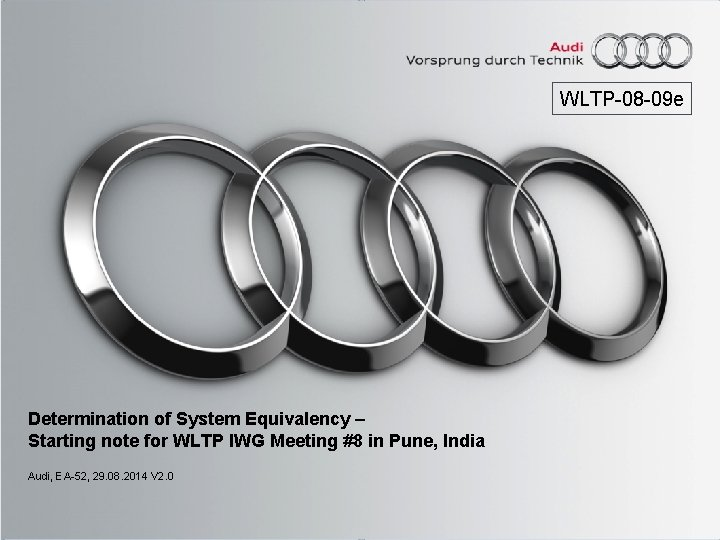WLTP-08 -09 e Determination of System Equivalency – Starting note for WLTP IWG Meeting #8 in Pune, India Audi, EA-52, 29. 08. 2014 V 2. 0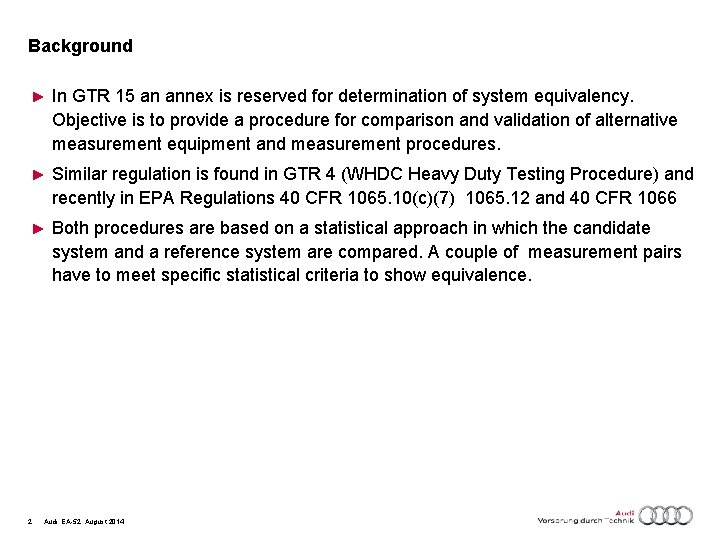Background ► In GTR 15 an annex is reserved for determination of system equivalency. Objective is to provide a procedure for comparison and validation of alternative measurement equipment and measurement procedures. ► Similar regulation is found in GTR 4 (WHDC Heavy Duty Testing Procedure) and recently in EPA Regulations 40 CFR 1065. 10(c)(7) 1065. 12 and 40 CFR 1066 ► Both procedures are based on a statistical approach in which the candidate system and a reference system are compared. A couple of measurement pairs have to meet specific statistical criteria to show equivalence. 2 Audi, EA-52, August 2014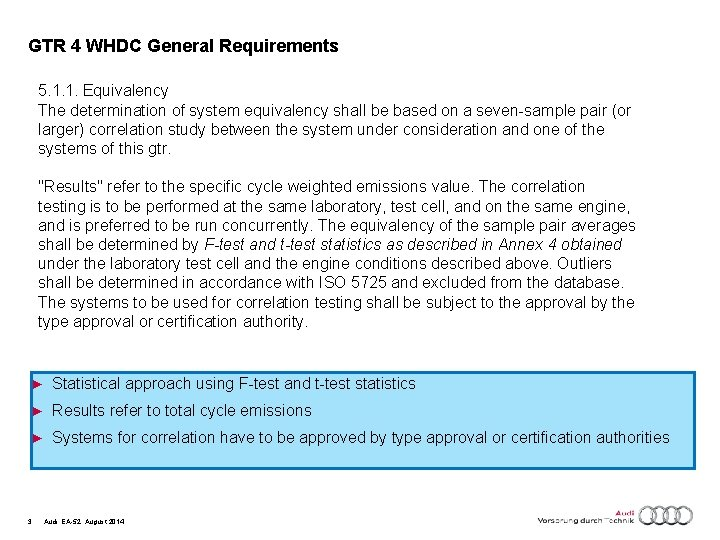GTR 4 WHDC General Requirements 5. 1. 1. Equivalency The determination of system equivalency shall be based on a seven-sample pair (or larger) correlation study between the system under consideration and one of the systems of this gtr. "Results" refer to the specific cycle weighted emissions value. The correlation testing is to be performed at the same laboratory, test cell, and on the same engine, and is preferred to be run concurrently. The equivalency of the sample pair averages shall be determined by F-test and t-test statistics as described in Annex 4 obtained under the laboratory test cell and the engine conditions described above. Outliers shall be determined in accordance with ISO 5725 and excluded from the database. The systems to be used for correlation testing shall be subject to the approval by the type approval or certification authority. ► Statistical approach using F-test and t-test statistics ► Results refer to total cycle emissions ► Systems for correlation have to be approved by type approval or certification authorities 3 Audi, EA-52, August 2014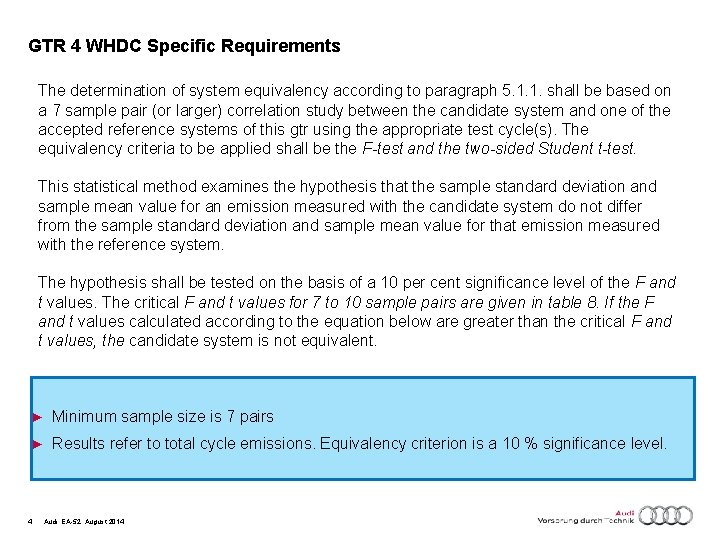GTR 4 WHDC Specific Requirements The determination of system equivalency according to paragraph 5. 1. 1. shall be based on a 7 sample pair (or larger) correlation study between the candidate system and one of the accepted reference systems of this gtr using the appropriate test cycle(s). The equivalency criteria to be applied shall be the F-test and the two-sided Student t-test. This statistical method examines the hypothesis that the sample standard deviation and sample mean value for an emission measured with the candidate system do not differ from the sample standard deviation and sample mean value for that emission measured with the reference system. The hypothesis shall be tested on the basis of a 10 per cent significance level of the F and t values. The critical F and t values for 7 to 10 sample pairs are given in table 8. If the F and t values calculated according to the equation below are greater than the critical F and t values, the candidate system is not equivalent. ► Minimum sample size is 7 pairs ► Results refer to total cycle emissions. Equivalency criterion is a 10 % significance level. 4 Audi, EA-52, August 2014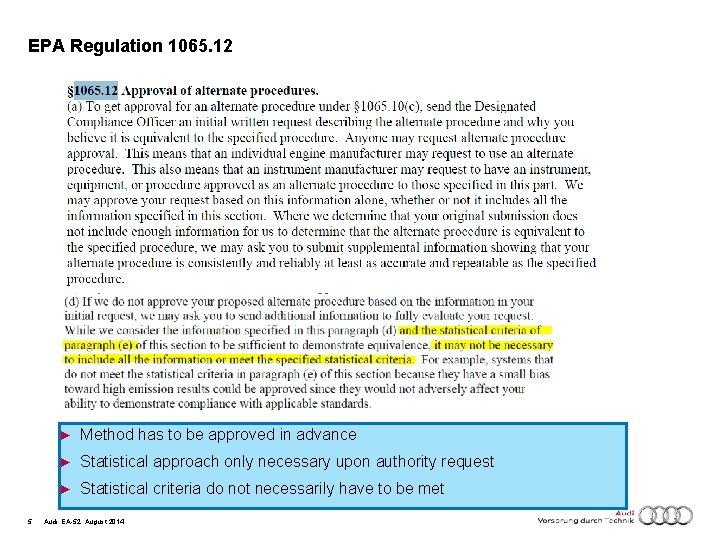EPA Regulation 1065. 12 5 ► Method has to be approved in advance ► Statistical approach only necessary upon authority request ► Statistical criteria do not necessarily have to be met Audi, EA-52, August 2014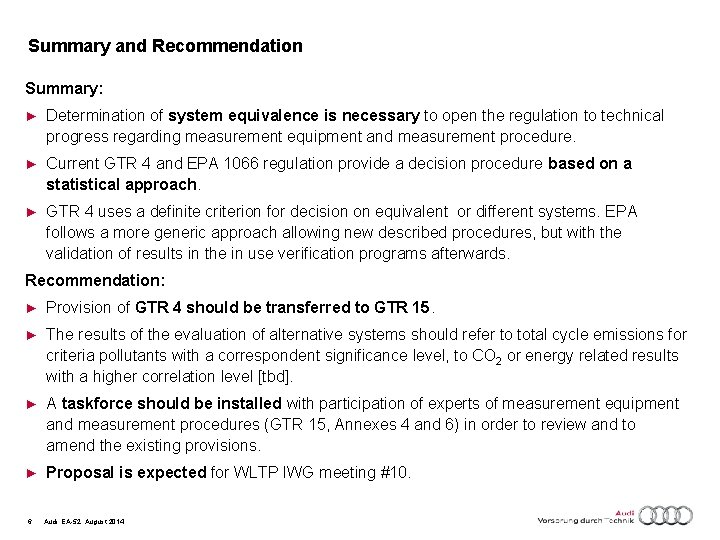Summary and Recommendation Summary: ► Determination of system equivalence is necessary to open the regulation to technical progress regarding measurement equipment and measurement procedure. ► Current GTR 4 and EPA 1066 regulation provide a decision procedure based on a statistical approach. ► GTR 4 uses a definite criterion for decision on equivalent or different systems. EPA follows a more generic approach allowing new described procedures, but with the validation of results in the in use verification programs afterwards. Recommendation: ► Provision of GTR 4 should be transferred to GTR 15. ► The results of the evaluation of alternative systems should refer to total cycle emissions for criteria pollutants with a correspondent significance level, to CO 2 or energy related results with a higher correlation level [tbd]. ► A taskforce should be installed with participation of experts of measurement equipment and measurement procedures (GTR 15, Annexes 4 and 6) in order to review and to amend the existing provisions. ► Proposal is expected for WLTP IWG meeting #10. 6 Audi, EA-52, August 2014Backup 7 Audi, EA-52, August 2014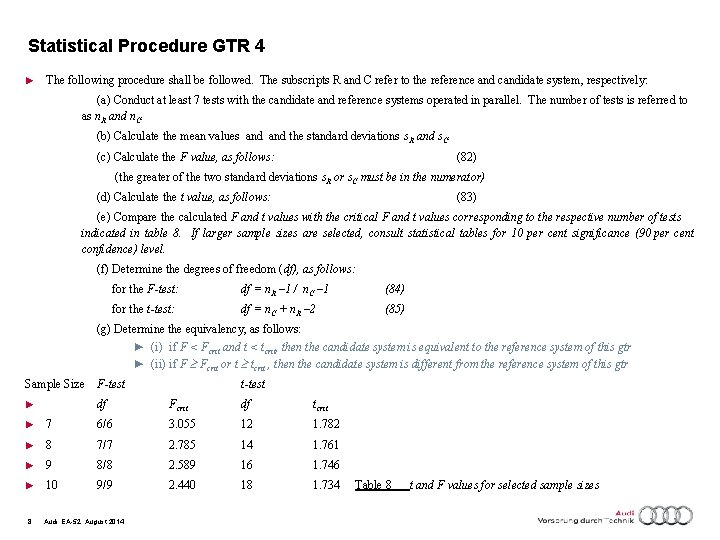Statistical Procedure GTR 4 ► The following procedure shall be followed. The subscripts R and C refer to the reference and candidate system, respectively: (a) Conduct at least 7 tests with the candidate and reference systems operated in parallel. The number of tests is referred to as n. R and n. C. (b) Calculate the mean values and the standard deviations s. R and s. C. (c) Calculate the F value, as follows: (82) (the greater of the two standard deviations s. R or s. C must be in the numerator) (d) Calculate the t value, as follows: (83) (e) Compare the calculated F and t values with the critical F and t values corresponding to the respective number of tests indicated in table 8. If larger sample sizes are selected, consult statistical tables for 10 per cent significance (90 per cent confidence) level. (f) Determine the degrees of freedom (df), as follows: for the F-test: df = n. R – 1 / n. C – 1 (84) for the t-test: df = n. C + n. R – 2 (85) (g) Determine the equivalency, as follows: ► (i) if F < Fcrit and t < tcrit, then the candidate system is equivalent to the reference system of this gtr ► (ii) if F Fcrit or t tcrit , then the candidate system is different from the reference system of this gtr Sample Size F-test t-test ► df Fcrit df tcrit ► 7 6/6 3. 055 12 1. 782 ► 8 7/7 2. 785 14 1. 761 ► 9 8/8 2. 589 16 1. 746 ► 10 9/9 2. 440 18 1. 734 8 Audi, EA-52, August 2014 Table 8 t and F values for selected sample sizesMathematics (to be amended) F-Test ► In comparing two independent samples of size N 1 and N 2 the F Test provides a measure for the probability that they have the same variance. The estimators of the variance are s 12 and s 22. ► We define as test statistic their ratio T = s 12/ s 22, which follows an F Distribution with f 1= N 11 and f 2= N 2 -1 degrees of freedom. One can formulate the F test for three different hypotheses, defined by: ► Tables of the quantiles , etc. , of the F distribution can be found in the literature 9 Audi, EA-52, August 2014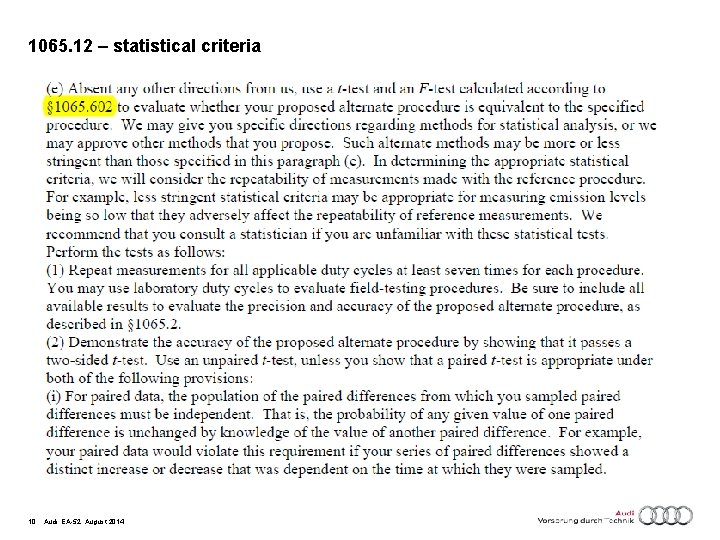1065. 12 – statistical criteria 10 Audi, EA-52, August 2014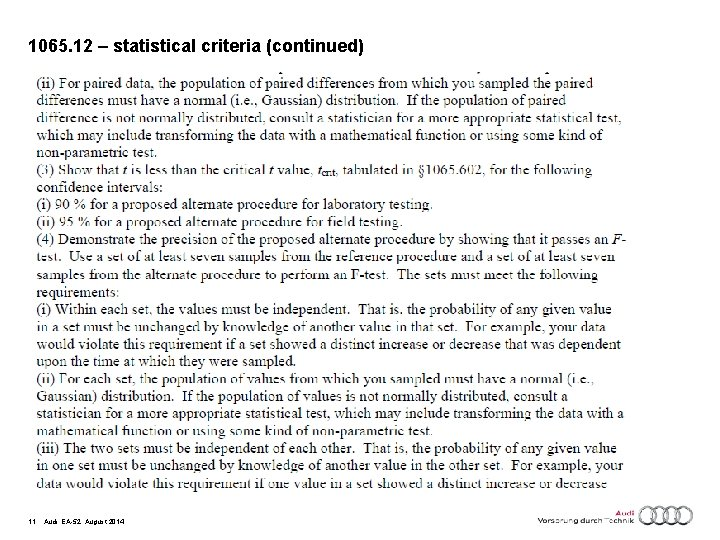1065. 12 – statistical criteria (continued) 11 Audi, EA-52, August 2014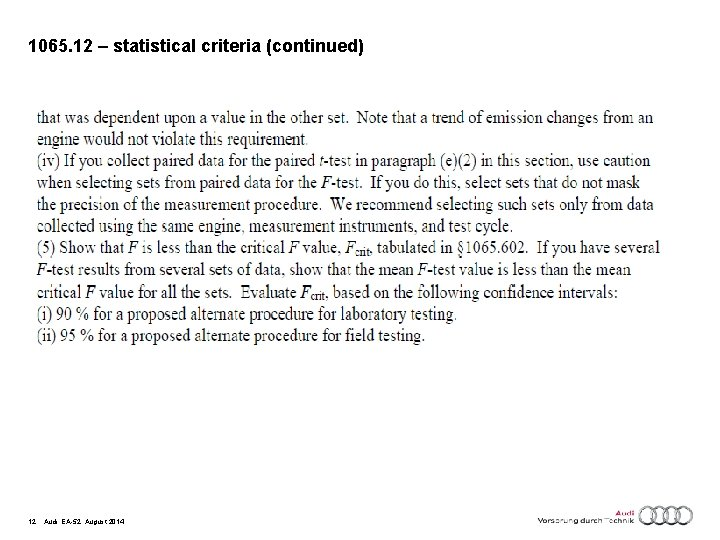1065. 12 – statistical criteria (continued) 12 Audi, EA-52, August 2014